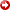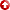[First Hit]# Integrating Functional Networks BasedNonlinearVariable Selection Methodology with Artificial Neural Networks for Improved Carbonate Reservoir Cementation Factor Prediction

## Abstract

An integrated machine learning workflow that combines thenonlinearvariable selection capability of functional networks (FNs) with the traditional feed-forward back-propagation algorithm of artificial neural networks (ANNs) is presented. The results of the proposed workflow (FN-ANN) are compared to multivariate linear regression (MLR), ANN fed with all input variables, and ANN fed with a subset of input variables selected based on linear correlation (LC-ANN). The proposed approach follows the hybrid machine learning paradigm. The FN algorithm extracts the best subset of input log variables based onnonlinearmapping with the reservoir cementation factor using the least square fitting criterion. The reservoir cementation factor, denoted by m in Archie’s equation, is obtained from special core analysis. The best subset of input logs extracted by the FN algorithm is used to build the proposed hybrid model to predict m for uncored wells. Datasets from five wells are used to build the model. Three other wells are used to validate the performance of the model. The performance of the proposed FN-ANN hybrid model is compared to MLR, ANN, and LC-ANN using the percentage match of the prediction curve with core data and statistical evaluation criteria. The overall results show that the FN-ANN outperformed the other models. For one of the test wells, the prediction curve produced by the FN-ANN model matches about 75% of the core data compared to the lower percentage match achieved by the other models. The statistical evaluation criteria (R-Square, p-value, and errors) agree with the percentage match. The FN-ANN model has the highest R-Square, and the lowest p-value and error. The results prove that the relationship between the input logs and m isnonlinear. It therefore confirms the efficiency of thenonlinearvariable selection process based on the FN algorithm. Therefore, m could not be reasonably estimated by using linear models such as MLR and LC-ANN. In addition to the improved accuracy indicated by the excellent match with the core data, the FN-ANN model also provides more cost-effective subset of variables for the prediction of m. Memory intensity is reduced especially when a very high-dimensional input space is involved. Other variable selection algorithms will be investigated in future work. This work fulfils the need for more accurate predictions of m, which is required for improved estimation of water saturation.